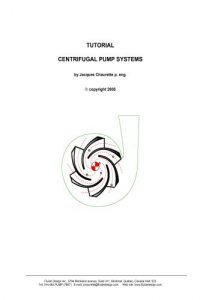اسم المؤلف
Jacques Chaurette
التاريخ
1 أغسطس 2021
المشاهدات
55
التقييم(لا توجد تقييمات)Loading...

Centrifugal Pump Systems Tutorial
by Jacques Chaurette p. eng.
Different types of pump systems

Three important characteristics of a pump system: pressure, friction and flow

What is friction in a pump system

Energy and head in pump systems

Flow rate depends on elevation difference or static head

Flow rate depends on friction

How does a centrifugal pump produce pressure

The performance or characteristic curve of the pump

How to select a centrifugal pump
Examples of total head calculations – sizing a pump for a home owner application

Examples of common residential water systems

Calculate the pump discharge pressure from the pump total head
Appendix A
Flow rate and friction loss for different pipe sizes based at different velocities
Appendix B
Formulas and an example of how to do pipe friction calculations
Appendix C
Formulas and an example of how to do pipe fittings friction calculations
Appendix D
Formula and an example of how to do velocity calculation for fluid flow in a pipe
Appendix E
The relationship between pressure head and pressure
كلمة سر فك الضغط : books-world.net